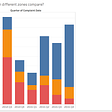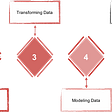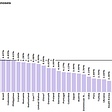# A Simple Trick to Understand the t-test

The t-test is a statistical test to compare the means of two groups. It is one of the most common techniques to check if two groups come from the same population. One of the most ubiquitous applications of the t-test in the industry is A/B testing, where two versions of UI are compared to determine which one generates more clicks or time spent on a page.

Unfortunately, understanding where the t-test’s equation comes from is a little bit tricky, especially given that there are many variants of the t-test out there, each serving its own purpose.

In this article, I will provide you with an intuition for the t-test’s equations using a simple signal-to-noise analogy.

If you look at the above variations of the t-test, first thing you’ll notice is that each formula is a fraction, with numerator being some sort of a difference between the two means. For example, one-sample t-test calculates the difference between the population’s mean (mu) and the sample’s mean (x). Independent two-sample t-test calculates the difference between two means of two samples — X1 and X2. We will call this difference a signal.

The denominator of the t-test’s equations represents the level of noise. To simplify, we can think of it as a level of variance between our samples (or sample and population). Higher values will correspond to more variance, and, as a result, more noise. Each of the denominators contains the sample’s standard deviation (s) with some combination of n. Together they form the standard error, which represents how spread out the numerator (signal) is expected to be.

Taken together, the signal/noise ratio represent the strength of the signal (difference of means), when the noise is taken into account. As the noise goes up and the signal goes down, the ratio goes down. If you’ve ever tried to have a conversation with someone on a rock concert you will understand what I mean. On the flip side, even barely audible whisper will sound very clear in a complete silence.

With this analogy in mind, it is straightforward to see under which conditions we can expect significant results for a t-test. In general, the higher your signal and the lower your noise — the lower the p-value (higher the absolute value of t-statistic). To illustrate this, below I generated two pairs of normally distributed samples with the fixed means (12 and 14), but different sample sizes and standard deviations.

I kept the signal constant at -2, however, the noise was set higher for the 2nd pair, as it depends on the sample size and standard deviation: smaller sample sizes and higher spread produce higher noise, which diminishes the signal. As a result, the t-statistic is significant for the 1st pair, but not significant for the 2nd pair.

Consider an extreme example of this relationship below:

With large sample size and small standard deviation, the noise is almost nil. As a result, any tiny difference between the means will be detectable. Graphically, it looks like the two samples do not overlap much. The resultant t-statistic is highly statistically significant, meaning the probability that the two samples come from the same population is negligible.

--

--

--

## More from Miroslav Tushev

CS PhD @ LSU. Passionate about statistics, ML, and NLP.

Love podcasts or audiobooks? Learn on the go with our new app.

## HCDE Sprint 8: Visualization## The 5 P’s of Data Science Projects## Notes On Anomaly Detection #2: Full Fat or Low-Calorie Fraud?## Ending The Year Planning For The Next## Covid19 Data for May 12, 2020## Spatial Autocorrelation: Close Objects Affecting Other Close Objects## Demystify p-value, α and Degree of Freedom in Statistics using Examples## Miroslav Tushev

CS PhD @ LSU. Passionate about statistics, ML, and NLP.

## Why is Chi-Squared Everywhere?## What is geometric average and why should we use it?## The schools of 💭 in Probability — Frequentist and Bayesian## Frequentist v/s Bayesian explained simply